# Use index and match instead of Vlookup

Most people who know Advanced Excel deal with the Vlookup function. This function is used to call data from a database. Suppose you have a list of people who have been registered with the information of individuals. And you are going to call in various places by entering the amount of the staffing number of each person’s personal information (including name, last name, etc.). For this purpose, the information is first recorded in a sheet or table. As in the following figure: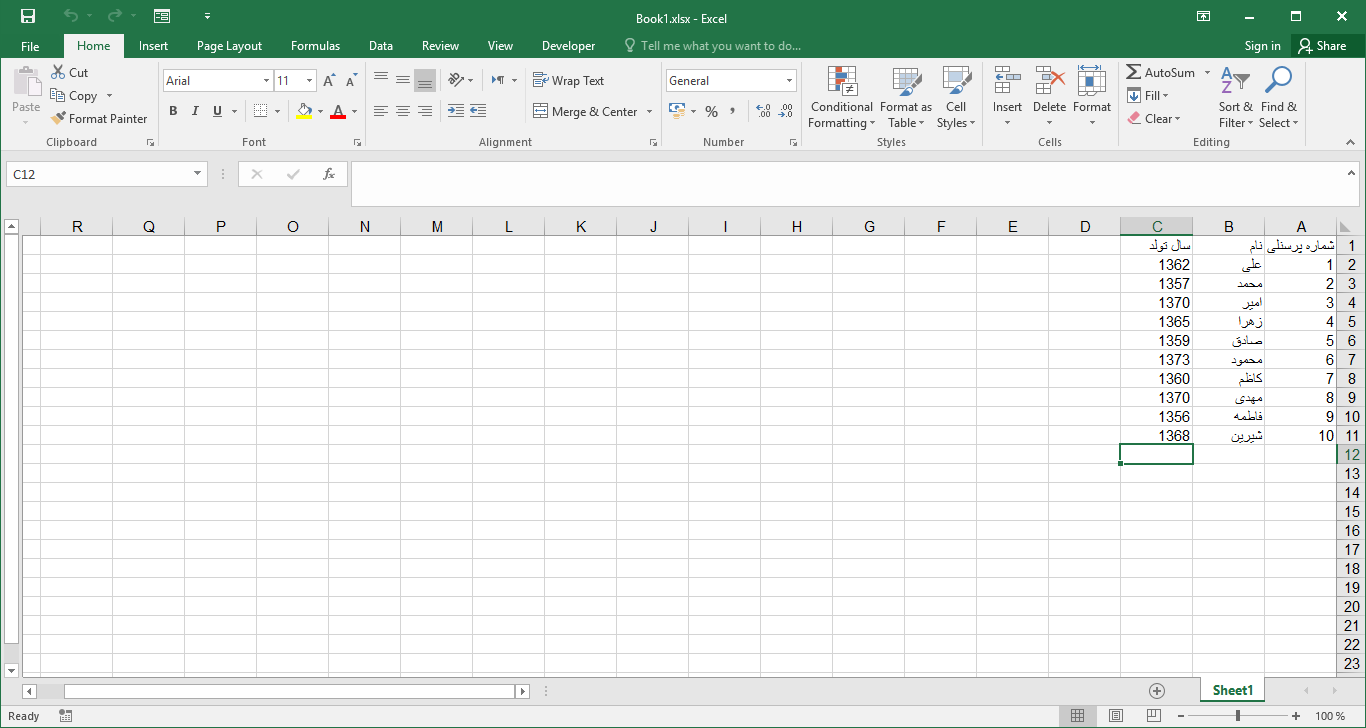Then, in the place where we need to call the information, we use the Vlookup function to call the value. Suppose, in the example above, suppose there is an information entry form like the one below that we want to enter the number of “name” and “year of birth” automatically by entering the personnel number: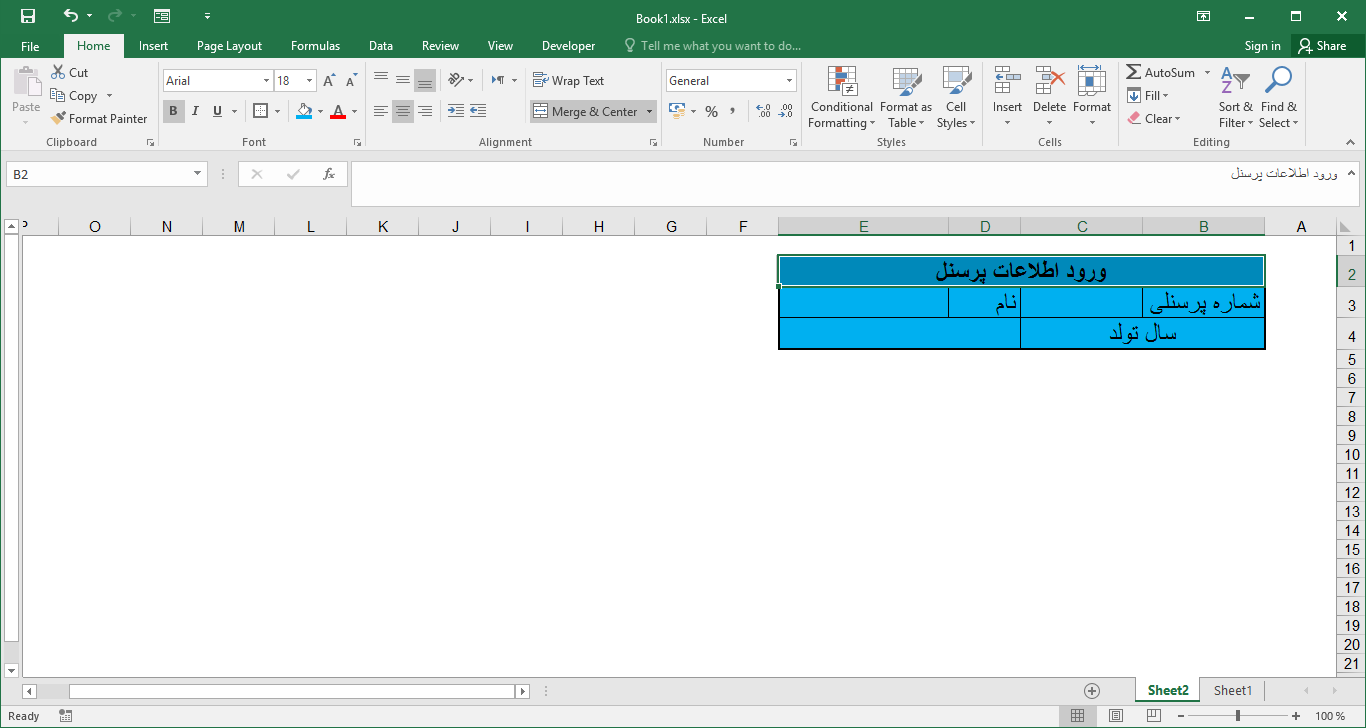For this purpose, we use the Vlookup function in the cell associated with the name. To do this in the cell, first write the sign “=” (start the formulation), and then write the Vlookup statement and complete the function arguments as below. The first argument (Lookup_value) is equal to the value we want to search for it (here the staff number), the second argument (Table_array) is the total of the range where the base information is located (here the first is the first argument), the third argument ( Col_index_num) is the number of the column that we want to call its value (here the “name” is in the second column, then the value “2”), the fourth argument is optional and its entry is not required and for the time being we do not have it so there is no need to enter that’s not.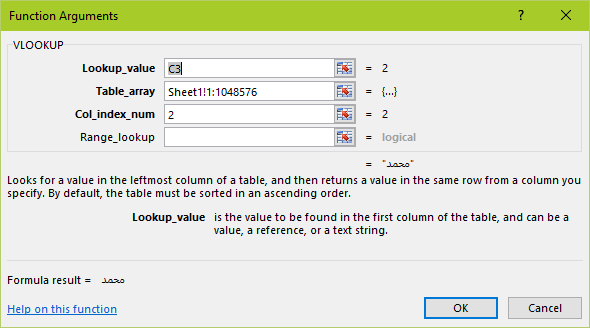Now entering the personnel number, the name is automatically completed: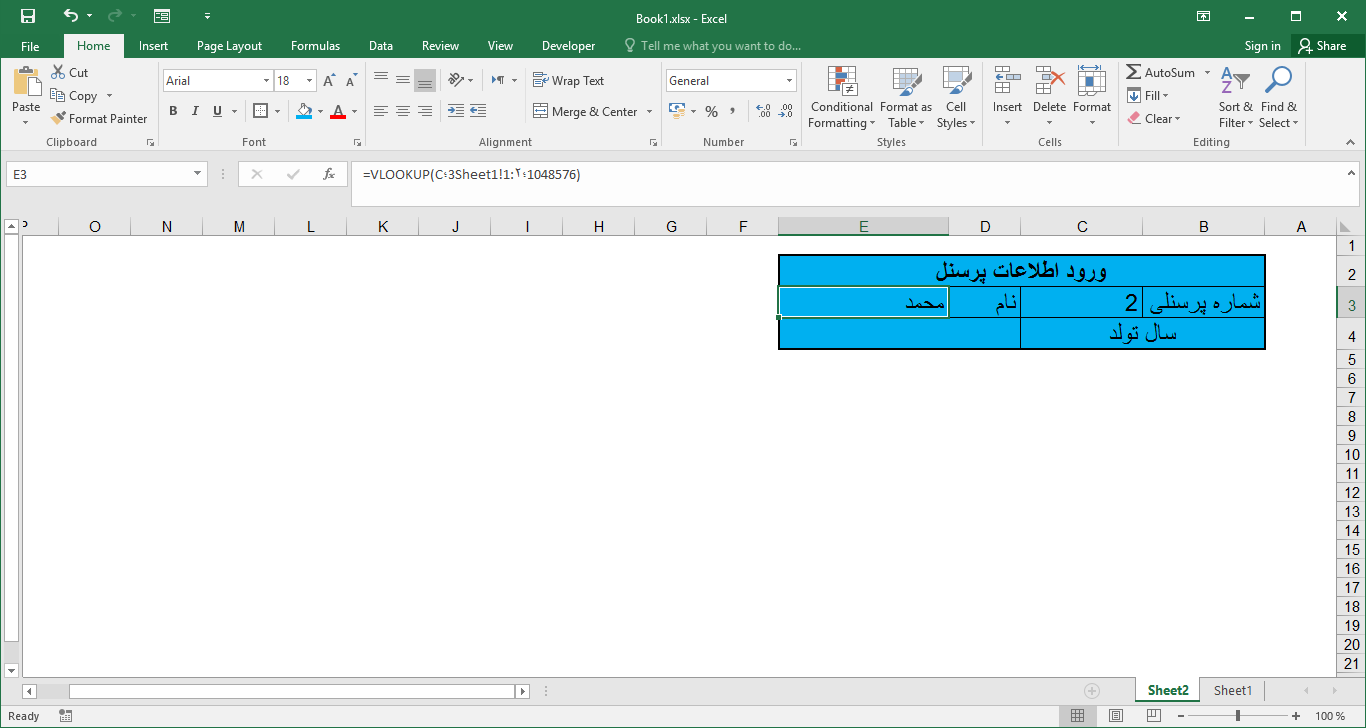The above steps worked with the Vlookup function, now we can do the same thing by combining the index and match functions. The reason is the higher speed of these functions than vlookup, as well as the fact that sometimes vlookup has limitations. (An example of these restrictions is described in this post).

Now we use the combination of index and match functions to complete the year of birth. First, for general understanding, keep in mind that the index function has 3 arguments, which takes the first argument of a range (containing several rows and columns), the second argument number of the row (in the range specified in the previous section), and the third argument number The column returns the value corresponding to the row number and column entered from within the range. For example, row 3 and column 2 from within range A1 to E13

The match function also finds a certain value in a specified range and returns its position (for example, its row number).

According to the above description to find the year of birth proportional to the number of personnel, we must first use the function function to match the row number corresponding to the number of personnel entered: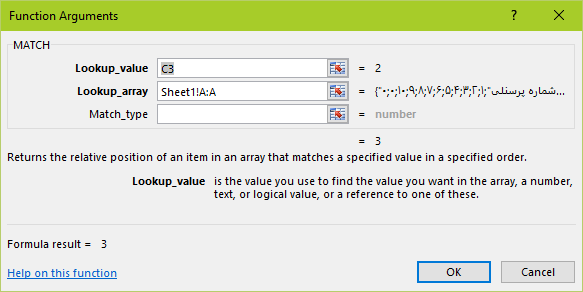Then, using the obtained upper row, we use the index function to call the given value (the second argument of the index function matches the value of the match function):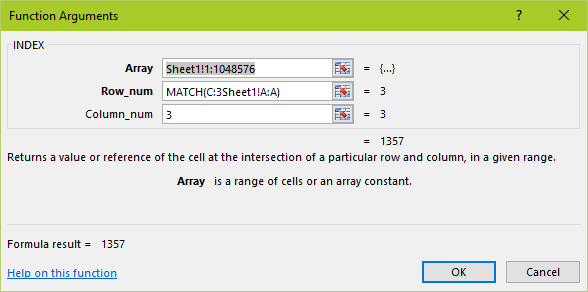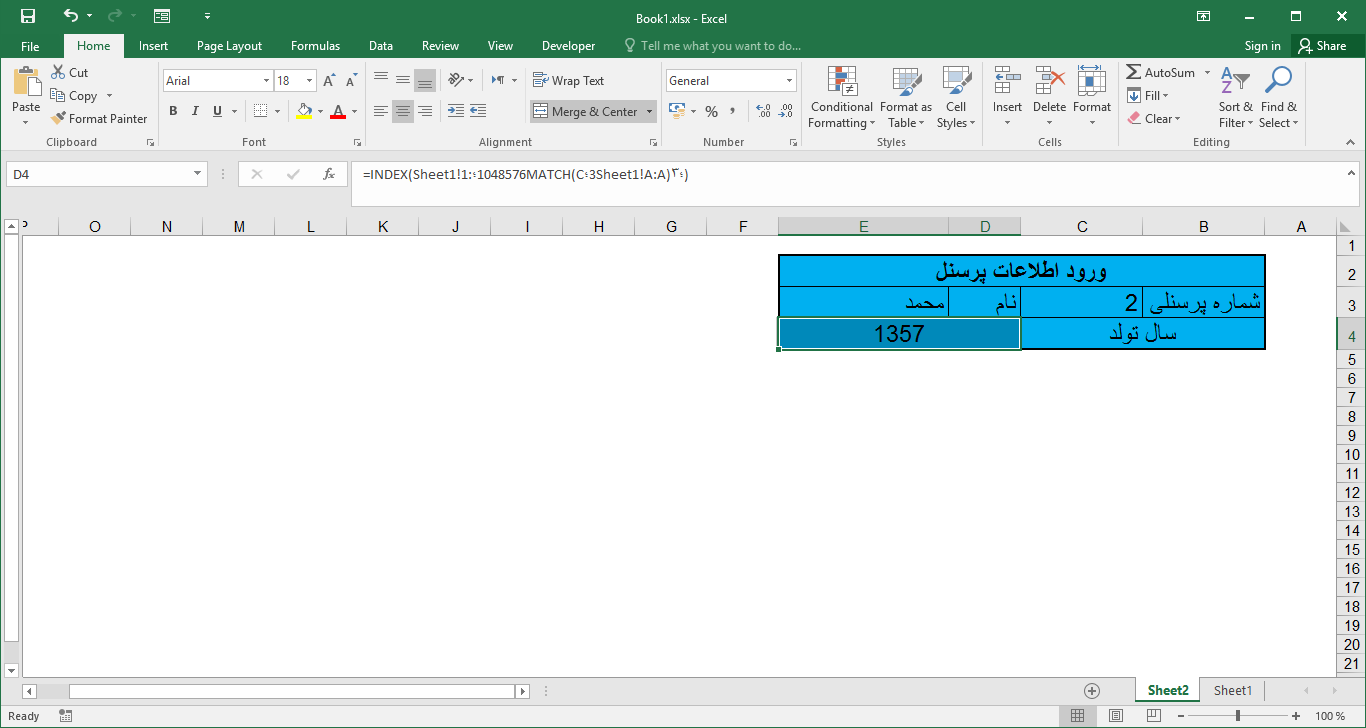Now it’s turning out to be a very interesting feature that is not possible with the Vlookup function (or at least I do not know! If anyone knows, thank you). Suppose we want 2 properties simultaneously checked, and if they have either 2 properties, then call them the appropriate value. For example, suppose that if the first column is equal to a and the second column is A, it will call the value corresponding to the third column! Suppose we want to call the amount of “year of production” corresponding to the year “1390” and the name “Ahmad”. For this purpose, we are similar to the previous one, but with the difference in how we use the formula formula. The match function is completed as follows: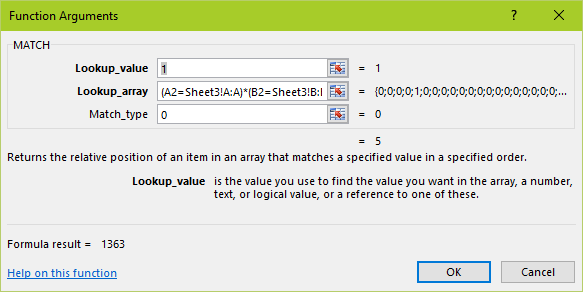In the above figure, as is clear, in the first argument the number is “1”, which means True, and in the second argument there is a conditional condition in which two conditions are simultaneously checked (these two conditions are the same 2) The above is said to be the year of production equal to 1390 and the equal name of Ahmad), and in the third argument, the number is 0. In this function it is checked and if the value of the entered clause in the second argument is True or “1”, the proportional value (row number) is returned! Note that if the number of conditions is more than 2, then similarly, it is possible to use this method.

The only thing to keep in mind is that with such a process, the amount returned is cellular and should be used for the correct representation of the final formula after Ctrl + Shift + Enter instead of Enter, which is done by doing this in Formula Load the total amount of the formula in the {} bracket.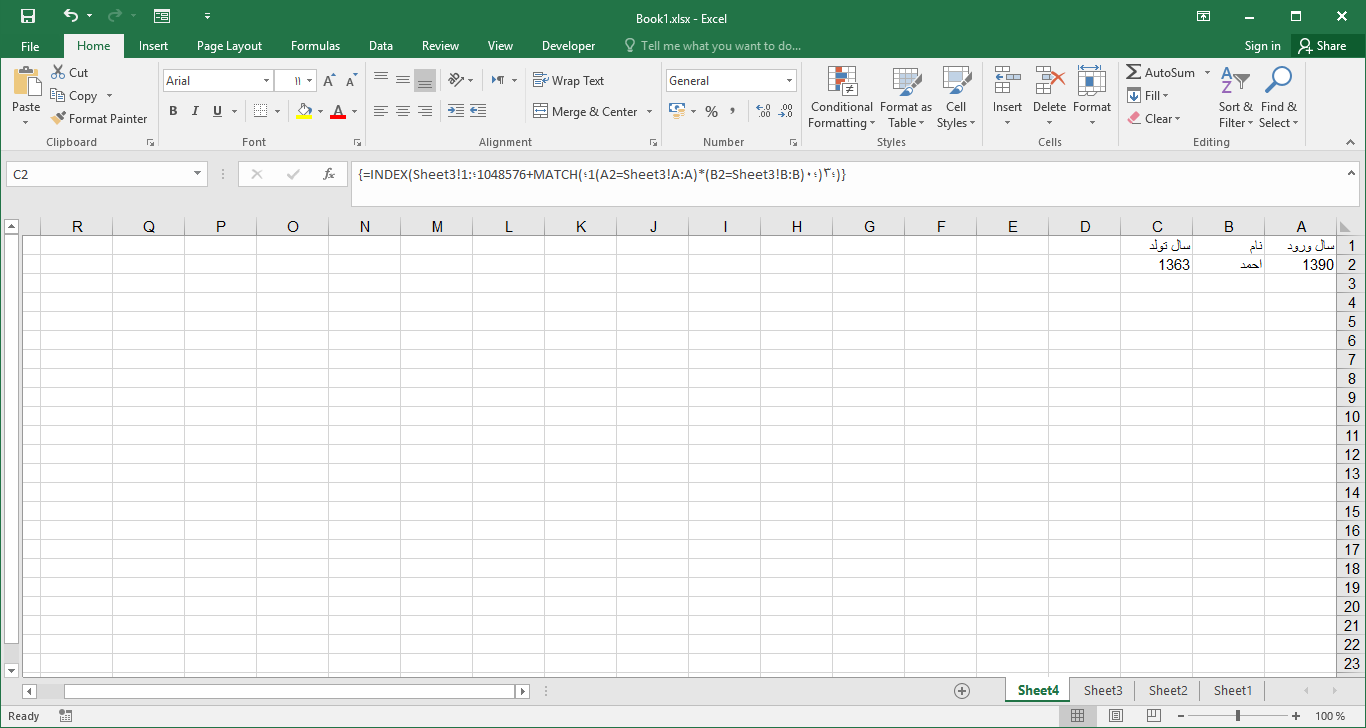An example sample file can also be downloaded from the following link: (Note that the screens 1 and 2 refer to the first step of the example, and the Shell 3 and 4 for the last one

Top